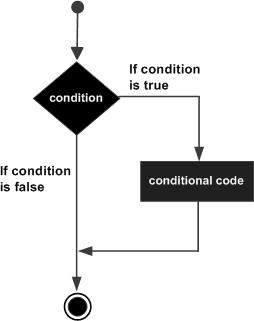## Pascal - if-then Statement

Contents[Hide]

The if-then statement is the simplest form of control statement, frequently used in decision making and changing the control flow of the program execution.

## 1. Syntax:

Syntax for if-then statement is:

```if condition then S
```

Where condition is a Boolean or relational condition and S is a simple or compound statement. Example of an if-then statement is:

```if (a =20) then
c := c+1;
```

If the boolean expression condition evaluates to true then the block of code inside the if statement will be executed. If boolean expression evaluates to false then the first set of code after the end of the if statement (after the closing end;) will be executed.

Pascal assumes any non-zero and non-nill values as true and if it is either zero or nill then it is assumed as false value.

## 2. Flow Diagram:## 3. Example:

Let us try a complete example that would illustrate the concept:

```program ifChecking;
var  {local variable declaration }
a:integer;
begin
a:=10;
(* check the boolean condition usingif statement *)
if( a <20) then
(*if condition istruethenprint the following *)
writeln('a is less than 20 ');
writeln('value of a is : ', a);
end.
```

When the above code is compiled and executed, it produces following result:

```a is less than 20
value of a is : 10

```